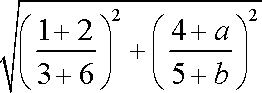# Elementary Algebra Syllabus Fall '07

INSTRUCTOR:  Geoff Hagopian
Email: ghagopian@collegeofthedesert.edu
WEB SITE: http://faculty.collegeofthedesert.edu/ghagopian
PHONE: 776-7223

# MPSetEqnAttrs('eq0001','',3,[[158,55,12,-1,-1],[211,74,16,-2,-2],[262,93,20,-2,-2],[],[],[],[659,229,49,-5,-5]]); MPEquation();COURSE DESCRIPTION: This course is an introduction to the real number system and to the use of variable expressions and equations in problem solving.  Topics include properties of the real numbers, square roots, arithmetic of variable expressions including polynomials and algebraic fractions, solving linear equations and inequalities in one variable, factoring, and an introduction to the Cartesian coordinate system and the equations and graphs of linear equations in two variables. Also, the proper use of math notation is emphasized along with an introduction to Pythagorean Theorem and basic geometric formulas, some dimensional analysis with modeling applications is included.

Topics delineated in the Course Outline

a.       Development of the Real Number System: Integers, Rationals, and Irrationals.

b.       Properties of Real Numbers and operations: commutative, associative, distributive, identity, inverse.

c.       The concept of a variable.

d.       Use of variables and algebraic method to generate algebraic expressions modeling an application problem.

e.       Arithmetic of algebraic expressions; the use of the commutative, associative, distributive, identity, and inverse properties, the use of the order of operations, and the use of integer exponents and the rules of exponents.

f.        Simplifying algebraic expressions, including algebraic fractions.

g.       Scientific notation

h.       Solving linear equations in one variable: addition, subtraction, multiplication, and division properties of equality

i.         Creating equations that model real world situations given in application (word) problems.

j.         Solving linear inequalities in one variable: addition, subtraction, multiplication, and division properties of inequality - One dimensional graphing of solutions.

k.       Creating inequalities that model real world situations given in word problems.

l.         Polynomial arithmetic, including multiplying polynomials.

m.     Factoring out the greatest common divisor from a polynomial expression.

o.       Solving rational equations that reduce to linear equations.

q.       The Cartesian coordinate system and the graphing of lines including the slope of a line and the different standard forms of the equation of a line.

TEXTBOOK: Understanding Elementary Algebra With Geometry, by Hirsch and Goodman.

LECTURE/HOMEWORK/LABS:  Generally, you can expect to learn more algebra applying yourself to the problems assigned for homework and lab time.  Of course, you will have a much easier time doing homework and performing well on exams if you pay attention and take excellent notes during lecture. Complete the homework assignment for each section to be well prepared for quizzes and exams. Do assignments to the best of your ability before the next class and have specific questions about places where you become stuck or confused.  You should be prepared at the beginning of each class with questions from the homework.  Homework notebooks will not be routinely collected; rather, I will ask to see your homework notebook from time to time and pose spot quizzes from the homework.

To get credit for homework assignments use the web site ilrn.com.  First find College of the Desert using their search and then

EXAMS:
There will be a tests after each chapter and a cumulative final exam. It is important to remember that the point of these exams is to test your understanding of the concepts covered; that is, they will not necessarily consist of just homework problems with the numbers changed, but may involve novel problems testing your understanding of how to use basic principles of calculus in context.

TECHNOLOGY:  A calculator is not required for this course and you will not be able to use a calculator on the tests.

ATTENDANCE:  You are required to attend all lecture and lab sessions: 6 hours per week for 16 weeks: a total of 96 hours.  The two most significant indicators for success in this course are attendance and completion of the homework. If you're not there doing what it is that defines the class, you missed it.

GRADE:  Your grade in this class will be a weighted average of your attendance, homework quiz, chapter test and final exam scores, using the following apportionment:

Attendance: 10 pts.
Homework/quiz: 10 pts.
Chapter tests: 50 pts.
Final Exam: 30 pts.